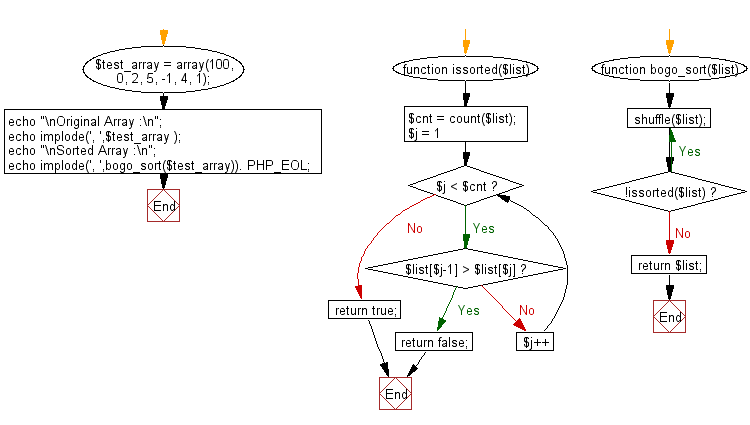# JavaScript Sorting Algorithm: Bogosort

## JavaScript Sorting Algorithm: Exercise-14 with Solution

Write a JavaScript program to sort a list of elements using Bogosort.

In computer science, bogosort is a particularly ineffective sorting algorithm based on the generate and test paradigm. The algorithm successively generates permutations of its input until it finds one that is sorted. It is not useful for sorting, but may be used for educational purposes, to contrast it with other more realistic algorithms.

Sample Solution: -

HTML Code:

``````<!DOCTYPE html>
<html>
<meta charset="utf-8">
<title>JavaScript program of Bogosort</title>
<body></body>
</html>
```
```

JavaScript Code:

``````function Bogosort(arr){
var isSorted = function(arr){
for(var i = 1; i < arr.length; i++){
if (arr[i-1] > arr[i]) {
return false;
}
}
return true;
};

function shuffle(arr){
var count = arr.length, temp, index;

while(count > 0){
index = Math.floor(Math.random() * count);
count--;

temp = arr[count];
arr[count] = arr[index];
arr[index] = temp;
}

return arr;
}

function sort(arr){
var sorted = false;
while(!sorted){
arr = shuffle(arr);
sorted = isSorted(arr);
}
return arr;
}

return sort(arr);
}

var arra = [3, 0, 2, 5, -1, 4, 1];
console.log("Original Array Elements");
console.log(arra);
console.log("Sorted Array Elements");
console.log(Bogosort(arra));
```
```

Sample Output:

```Original Array Elements
[3,0,2,5,-1,4,1]
Sorted Array Elements
[-1,0,1,2,3,4,5]
```

Flowchart:Live Demo:

See the Pen searching-and-sorting-algorithm-exercise-14 by w3resource (@w3resource) on CodePen.

Improve this sample solution and post your code through Disqus

What is the difficulty level of this exercise?

Test your Programming skills with w3resource's quiz.

﻿